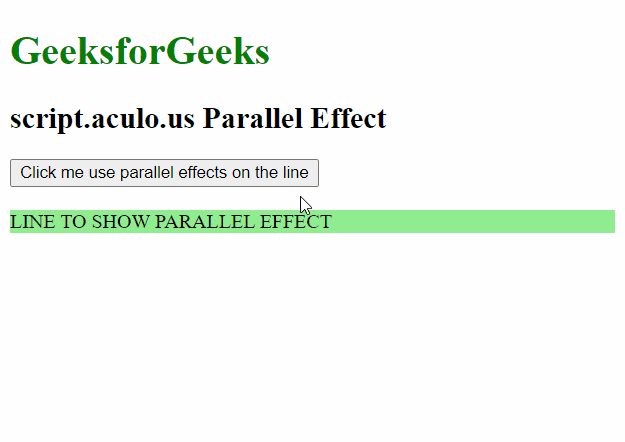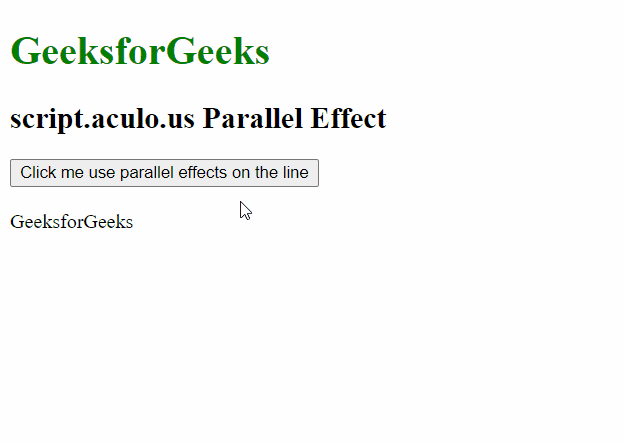Related Articles
script.aculo.us Parallel Effect
• Last Updated : 20 Nov, 2020

The script.aculo.us library is a cross-browser library that aims to improving the user interface of a website. In this article, we will demonstrate the Parallel effect. This effect is used for combining multiple effects for use on the given element. We can adjust the duration of the effect as well.

Syntax:

`Effect.Parallel( [array of subeffects], [options] )`

Parameters: This effect has a single parameter in the options object described below:

• sync: It is a boolean value that can prevent the effects from starting as soon as they are initialized, synchronizing them.

To demonstrate the use of this function, we have written a small piece of code. In which, we have written a small JavaScript function named ShowEffect method which uses the Parallel method of this library. The examples below demonstrate the method.

Example 1:

## HTML

 ```<``html``>`` ` `<``head``>``    ``<``script` `type``=``"text/javascript"` `        ``src``=``"prototype.js"``>``    ```` ` `    ``<``script` `type``=``"text/javascript"` `        ``src``=``"scriptaculous.js"``>``    ```` ` `    ``<``script` `type``=``"text/javascript"``>``        ``function ShowEffect(element) {`` ` `            ``// Using the Parallel effect``            ``// with 2 effects and default``            ``// options``            ``new Effect.Parallel([``                ``new Effect.Move(element, {``                    ``x: 20,``                    ``y: 50,``                    ``mode: 'relative'``                ``}),``                ``new Effect.Opacity(element, {``                    ``from: 1,``                    ``to: 0``                ``})``            ``]);``        ``}``    `````` ` `<``body``>``    ``<``h1` `style``=``"color: green;"``>``        ``GeeksforGeeks``    ```` ` `    ``<``h2``>script.aculo.us Parallel Effect``     ` `    ``<``button` `onclick``=``"ShowEffect('hideshow')"``>``        ``Click me use parallel ``        ``effects on the line``    ```` ` `    ``<``br``><``br``>``    ``<``div` `id``=``"hideshow"` `style``=``        ``"background-color: lightgreen;"``>``        ``LINE TO SHOW PARALLEL EFFECT``    `````` ` ``

Output:Example 2:

## HTML

 ```<``html``>`` ` `<``head``>``    ``<``script` `type``=``"text/javascript"` `        ``src``=``"prototype.js"``>``    ```` ` `    ``<``script` `type``=``"text/javascript"` `        ``src``=``"scriptaculous.js"``>``    ```` ` `    ``<``script` `type``=``"text/javascript"``>``        ``function ShowEffect(element) {`` ` `            ``// Using the Parallel effect``            ``// with 2 effects and the``            ``// sync parameter``            ``new Effect.Parallel([``                ``new Effect.Move(element, {``                    ``x: 100,``                    ``y: 50,``                    ``mode: 'relative'``                ``}),``                ``new Effect.SwitchOff(element)``            ``], {``                ``sync: false``            ``});``        ``}``    `````` ` `<``body``>``    ``<``h1` `style``=``"color: green;"``>``        ``GeeksforGeeks``    ```` ` `    ``<``h2``>script.aculo.us Parallel Effect``     ` `    ``<``button` `onclick``=``"ShowEffect('geeks_1')"``>``        ``Click me use parallel ``        ``effects on the line``    ````     ` `    ``<``br``><``br``>``    ``<``div` `id``=``"geeks_1"` `style``=``        ``"position: absolute"``>``        ``GeeksforGeeks``    `````` ` ``

Output:My Personal Notes arrow_drop_up